# Maths Division Questions For KidsDivision is another basic operation of simple Arithmetical problems. For new school goers, the division is a new mathematical concept in which the problem is broken down into a series of easy and simple steps. An obelus symbol (÷ or †) is used to represent the mathematical operation of division. Young learners are made to learn simple mathematical division sums at the elementary level.

Young kids are taught to solve simple and easy division questions with examples like how to divide cookies among themselves, divide single-digit and two-digit numbers, understand long division sums and solve basic Maths division questions like (10÷2 =5). Having a sound knowledge about division sums is an effective step towards kids learning as it prepares young children to gear up for higher classes.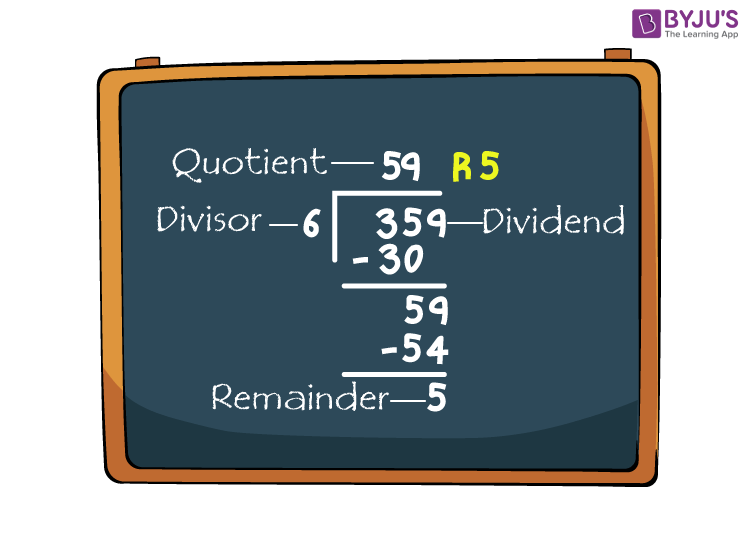In the image given above, there is a relationship between the various parts of division sums problem which are explained below –

• Dividend: It refers to the number which we divide. In the problem, the dividend is 359.
• Divisor: It refers to the number by which we divide, here the divisor is 6.
• Quotient: This is the result or outcome that we obtain from the problem. In this case, it is 59.
• Remainder: This is the remaining number that is left over after division, here the remainder is 5.

## What is Simple Division?

In Maths, the simple division is a method used for dividing small numbers into groups and parts. Simple division sums help in dividing easy and simple division questions into divisible terms. One such example of simple division questions is given in the image attached below: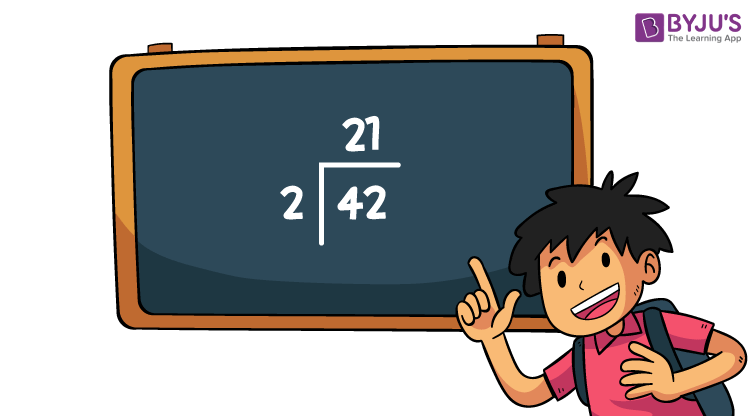Some more examples: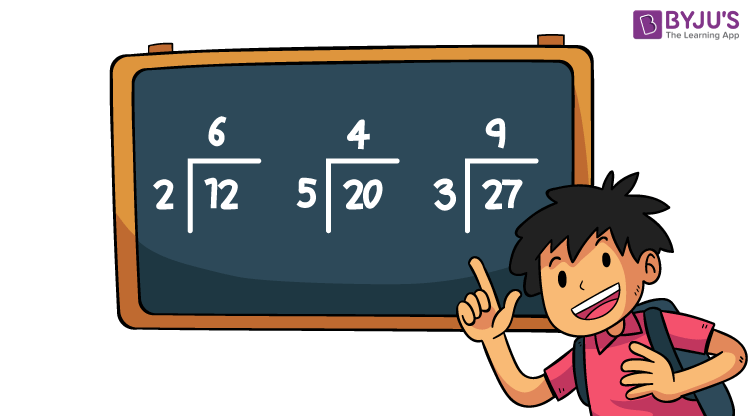## What is Long Division?

In Mathematics, long division is a method used for dividing large numbers into small groups or parts. It helps in breaking the long division sums problem into a sequence of simple and easier steps. Just like other long division questions, a large number (dividend) is divided by another number (divisor) which gives a result (quotient) and sometimes the number left over which is known as remainder.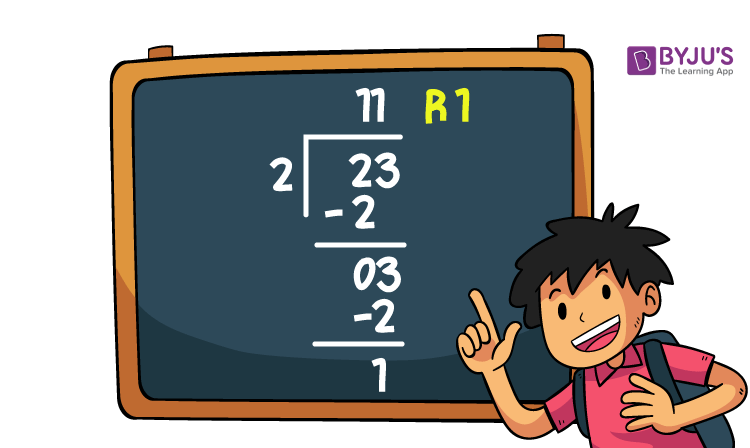Some more examples: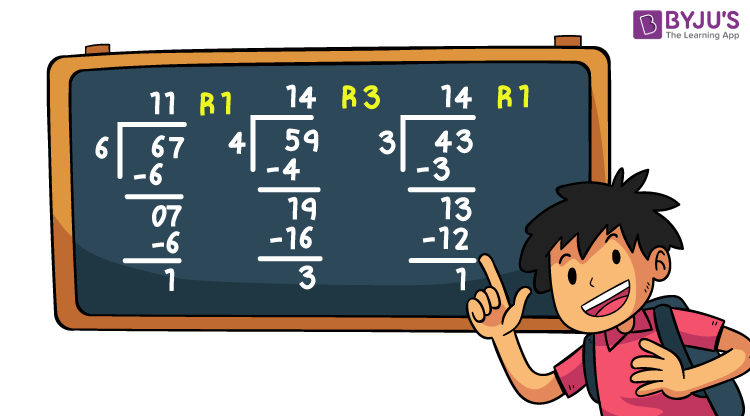Young learners are more receptive to learning new things every day. Some division questions include dividing a single or double-digit number, three-digit calculation and long division questions, etc. Meanwhile, you can also download the Disney BYJU’S Early Learn App and make learning an entertaining and fun-filled experience for your child.